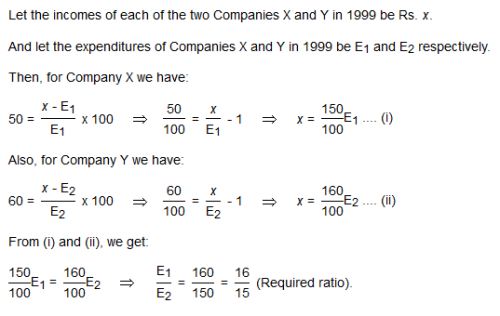Courses

# Test: Line Charts- 4

## 5 Questions MCQ Test General Aptitude for GATE 2020 | Test: Line Charts- 4

Description
This mock test of Test: Line Charts- 4 for LR helps you for every LR entrance exam. This contains 5 Multiple Choice Questions for LR Test: Line Charts- 4 (mcq) to study with solutions a complete question bank. The solved questions answers in this Test: Line Charts- 4 quiz give you a good mix of easy questions and tough questions. LR students definitely take this Test: Line Charts- 4 exercise for a better result in the exam. You can find other Test: Line Charts- 4 extra questions, long questions & short questions for LR on EduRev as well by searching above.
QUESTION: 1

### The following line graph gives the percent profit earned by two Companies X and Y during the period 1996 - 2001.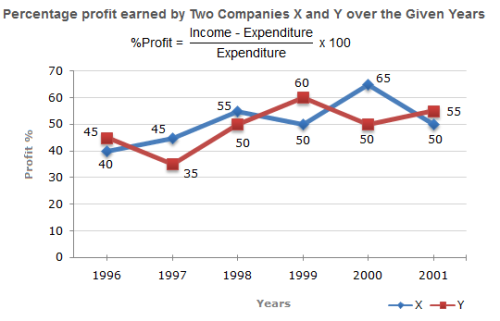The incomes of two Companies X and Y in 2000 were in the ratio of 3:4 respectively. What was the respective ratio of their expenditures in 2000 ?

Solution: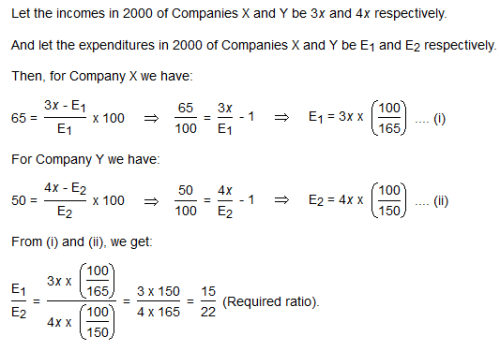QUESTION: 2

###If the expenditure of Company Y in 1997 was Rs. 220 crores, what was its income in 1997 ?

Solution: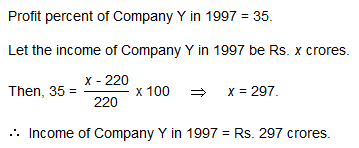QUESTION: 3

### The following line graph gives the percent profit earned by two Companies X and Y during the period 1996 - 2001.If the expenditures of Company X and Y in 1996 were equal and the total income of the two Companies in 1996 was Rs. 342 crores, what was the total profit of the two Companies together in 1996 ? (Profit = Income - Expenditure)

Solution: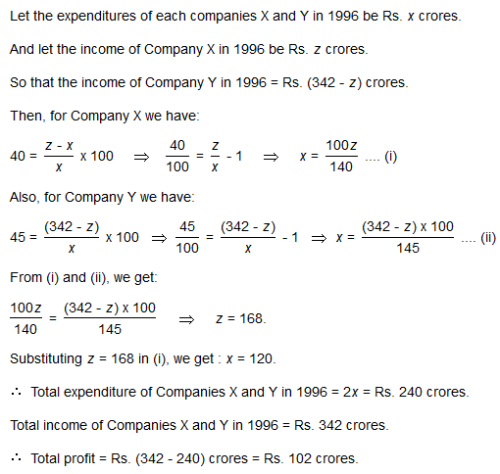QUESTION: 4

The following line graph gives the percent profit earned by two Companies X and Y during the period 1996 - 2001.The expenditure of Company X in the year 1998 was Rs. 200 crores and the income of company X in 1998 was the same as its expenditure in 2001. The income of Company X in 2001 was ?

Solution: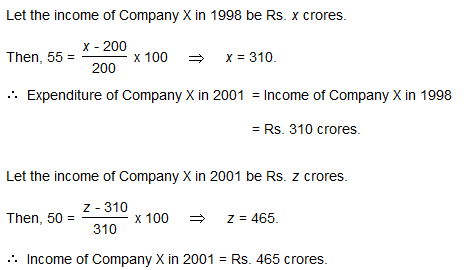QUESTION: 5

The following line graph gives the percent profit earned by two Companies X and Y during the period 1996 - 2001If the incomes of two Comapanies were equal in 1999, then what was the ratio of expenditure of Company X to that of Company Y in 1999 ?

Solution: